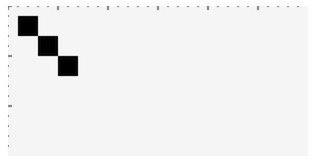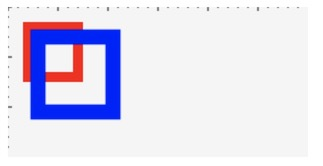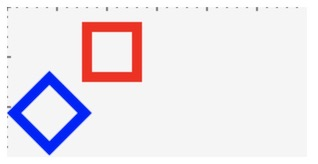CSS3中有一个很重要的点，就是形变。他分为移动，缩放、旋转和倾斜。在Canvas中，形变都是基于坐标做的，所以，并没有直接的API支持倾斜，其它几种都是有独立的API来支持，命名和CSS是一样的。今天我们就看一下这几种吧。

## 平移## 缩放## 旋转## 矩阵变换

$$\left[ \begin{matrix} a & c & e \\ b & d & f \\ 0 & 0 & 1 \end{matrix} \right]$$

$$x_1 = x_0 + e \\ y_1 = y_0 + f$$

$$\left[ \begin{matrix} x_1 \\ y_1 \\ 1 \\ \end{matrix} \right] = \left[ \begin{matrix} 1 & 0 & e \\ 0 & 1 & f \\ 0 & 0 & 1 \end{matrix} \right] \left[ \begin{matrix} x_0 \\ y_0 \\ 1 \\ \end{matrix} \right]$$

$$x_1 = a * x_0 \\ y_1 = d * y_0$$

$$\left[ \begin{matrix} x_1 \\ y_1 \\ 1 \\ \end{matrix} \right] = \left[ \begin{matrix} a & 0 & 0 \\ 0 & d & 0 \\ 0 & 0 & 1 \end{matrix} \right] \left[ \begin{matrix} x_0 \\ y_0 \\ 1 \\ \end{matrix} \right]$$

$$x_1 = x_0 * cos\theta - y_0 * sin\theta \\ y_1 = x_0 * sin\theta + y_0 * cos\theta$$

$$\left[ \begin{matrix} x_1 \\ y_1 \\ 1 \\ \end{matrix} \right] = \left[ \begin{matrix} cos\theta & -sin\theta & 0 \\ sin\theta & cos\theta & 0 \\ 0 & 0 & 1 \end{matrix} \right] \left[ \begin{matrix} x_0 \\ y_0 \\ 1 \\ \end{matrix} \right]$$

-------------本文结束 感谢您的阅读-------------
0%